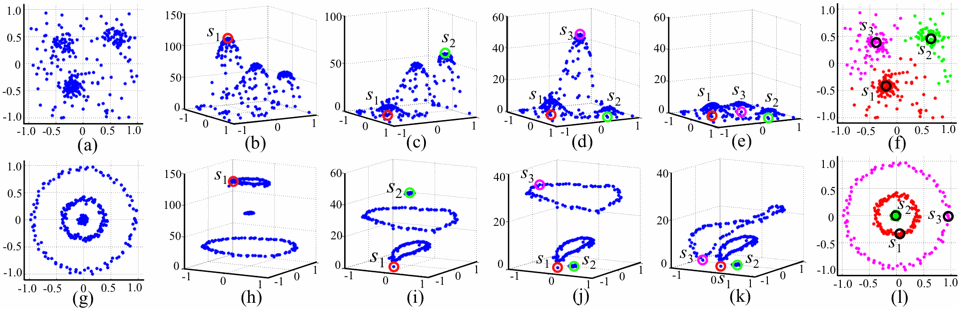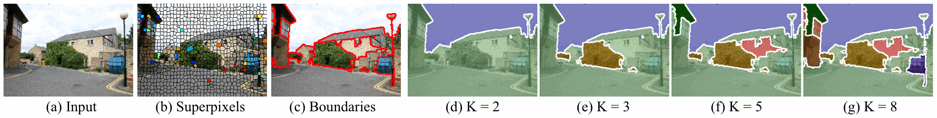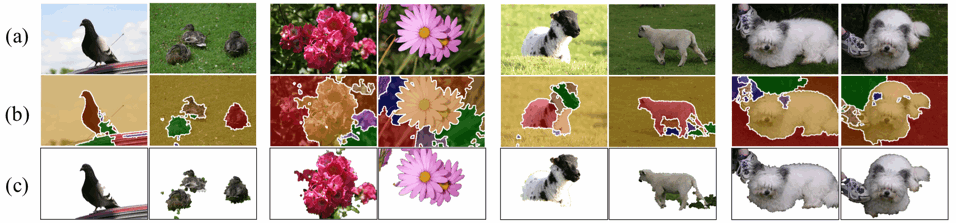# Diversity Ranking, Image Segmentation, and Cosegmentation via Submodular Optimization on Anisotropic Diffusion

Paper and Matlab toolbox are now available.

## Matlab code

1. Note that this toolbox was re-written for the instruction purpose. I will post benchmark code later.

2. (Last update: 08/28/2011) Please note that this beta toolbox may be frequently updated for a while.

3. Major update will be around end of October 2011.

2. If you need image segmentation, our toolbox requires a superpixel algorithm.

1. In our experiments, Turbopixel was the best. Download the Turbopixel here.

2. Copy the tar file to the 'lib’ subdirectory of our toolbox, and untar it.

3. Install Turbopixel by following its README.txt.

4. If you find any bugs, please email me.

## Description

The optimization problem of this paper is as follows; Given a system under anisotropic heat diffusion and finite K heat sources, where should one place all the sources in order to maximize the temperature of the system?

Our main theoretic result is to prove that the temperature under linear anisotropic diffusion is a submodular function; therefore, a simple greedy algorithm guarantees at least a constant factor approximation to the optimal solution for the above temperature maximization. This result is successfully applied to scalable cosegmentation as well as diversity ranking and single-image segmentation.

1. Example of Diversity Ranking

The following pictures show two toy examples of diversity ranking. The data points are randomly generated from three Gaussian distributions in (a) and three co-centric circles in (g). In (b)-(e) and (h)-(k), the marginal temperature gain of each point is shown along z-axis. The greedy algorithm iteratively selects s_k as the point with maximum marginal gain. Once a point is selected, the marginal gains of its neighbors largely drop because they already get high temperatures. In (f)(l), final three clusters are shown.2. Example of Single image segmentation

Our segmentation algorithm greedily selects the largest and most coherent regions. As shown in the following example, the sky is first chosen with K=2. As K increases, the regions of the tree, the house in the center, and the building in the left are chosen in the decreasing order of their sizes and coherence in (d)-(g). This behavior is quite helpful for automatic selection of K. We can keep increasing K until the detected segment is not significant any more (i.e. temperature increase by adding a new source is not significant any more).3. Example of cosegmentation.

The following figures show some examples of cosegmentation on the MSRC. We made two interesting observations here: (i) Our method can easily segment out multiple instances in the images. (ii) Our algorithm is robust against an incorrect selection of K. In the duck example of the second column, the best choice of K would be four, but a faulty guess with K=8 did little harm. The four significant segments are successfully detected (e.g.three ducks and grass) and the other four overestimated segments were trivially selected as tiny dots.## Funding

• NSF DBI 0640543, AFOSR FA 9550-10-1024, and ONR N000140910758 to Eric P. Xing.

• MURI N00014-07-1-0747 to Takeo Kanade.